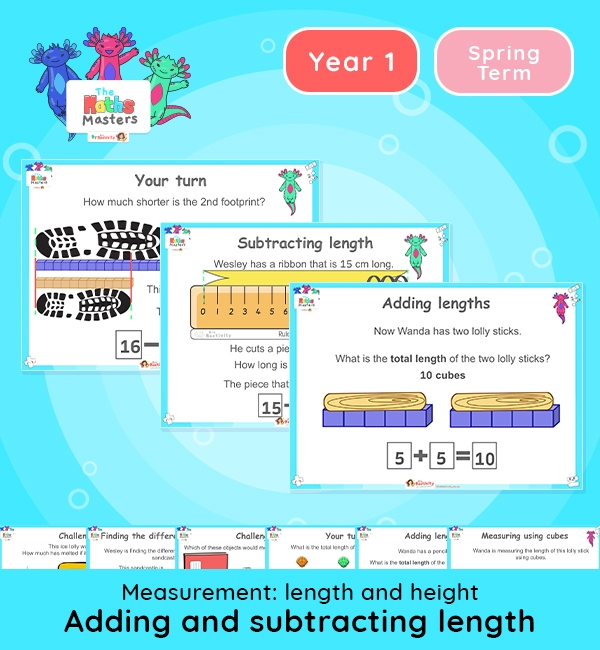# Year 1 | Adding and Subtracting Length Lesson Presentation## Year 1 adding and subtracting length resources

Topic: Measurement: length and height

This lesson focuses on adding and subtracting lengths and heights using standard and non-standard units. It is a longer presentation and may be best taught over 2 sessions – one on adding lengths and another on subtracting lengths.

NC Links: •Measure and begin to record lengths and heights •Compare, describe, and solve practical problems for lengths and heights (for example, long/short, longer/shorter. tall/short, taller/shorter). *Begin to use measuring tools such as a ruler.

*Solve one step problems that involve addition and subtraction.

TAF Statements: Working towards: Add and subtract one-digit numbers

Working At: Read scales in divisions of ones, twos, fives.

Greater depth: Use reasoning about number and relationship to solve more complex problems and explain their thinking.

Ready-to-progress criteria:  1AS-2 Read, write and interpret equations containing addition, subtraction and equals symbols and relate additive expression and equations to real life contexts.

Previous experience: Devise and record number stories using pictures, numbers and symbols.

## Recently Viewed